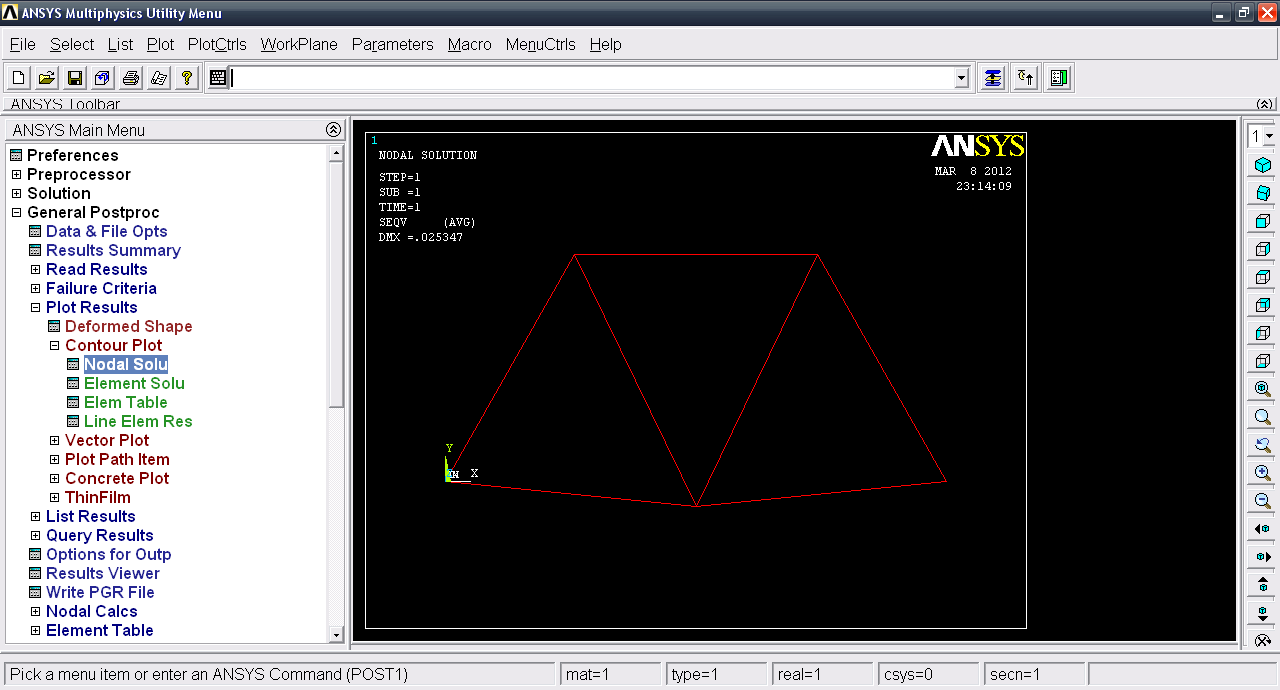# Tutorial - 2D Truss analysis in Mechanical APDL (ANSYS) Part 2?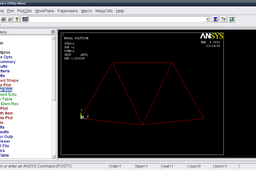In general, a finite element solution may be broken into the following three stages.
1. Preprocessing: defining the problem;
- Define keypoints/lines/areas/volumes
- Define element type and material/geometric properties
- Mesh lines/areas/volumes as required
2. Solution: assigning loads, constraints and solving;
3. Postprocessing:
- Lists of nodal displacements
- Element forces and moments
- Deflection plots
- Stress contour diagrams

In this tutorial we will go through second and third step.

1. ### Step 1:

This is the second part of tutorials in Which we solve the problem. Under Solution >> Analaysis Type >> New analysis. Select static and click OK.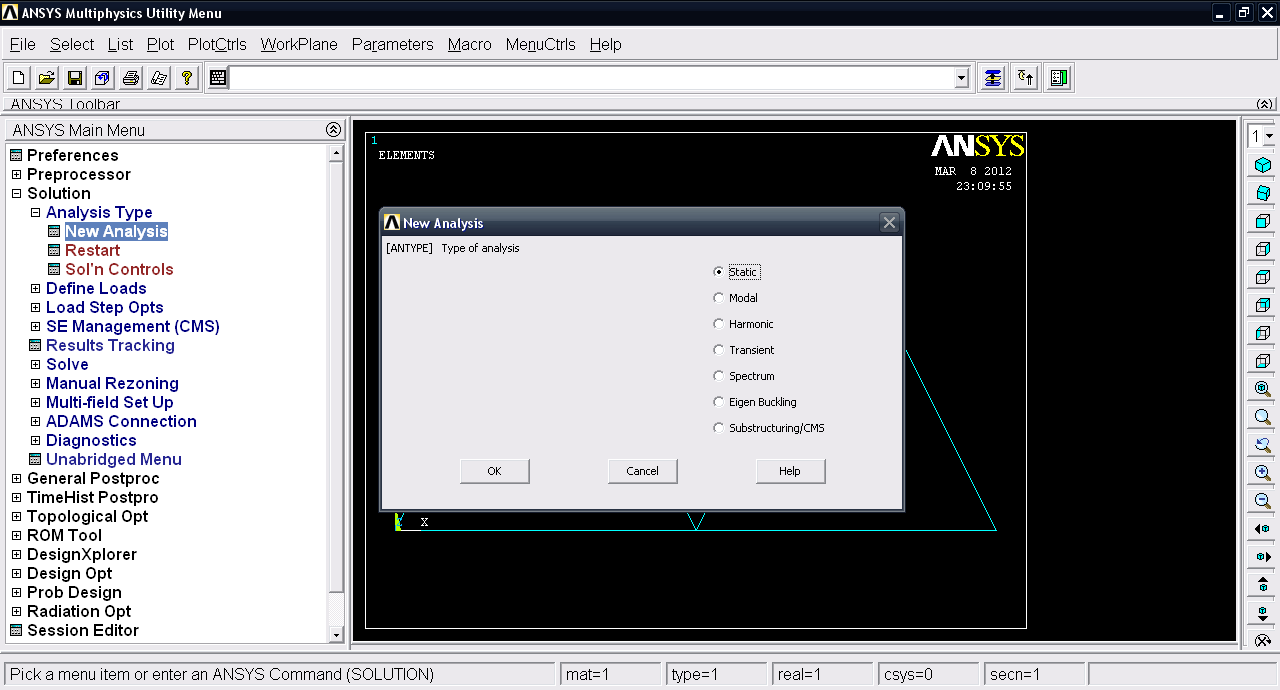2. ### Step 2:

Under Define Loads >> Apply >> Structural >> Displacement >> On keypoints. We will now define the fixed keypoints or supports.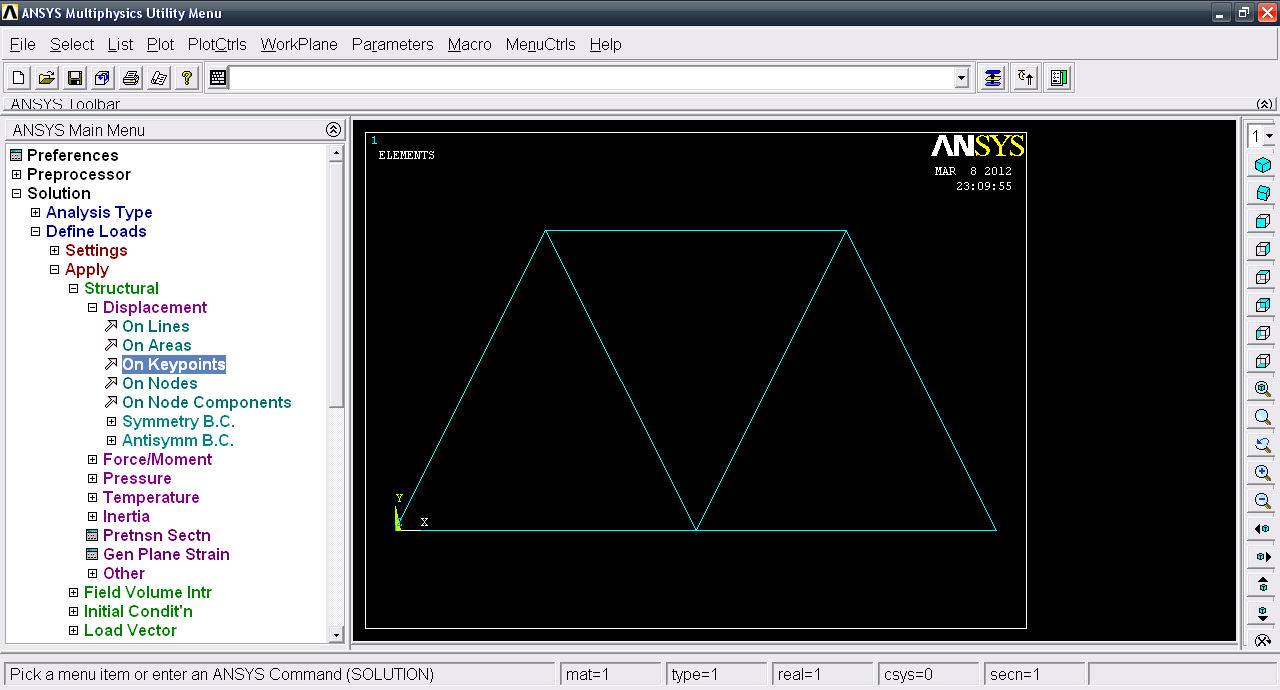3. ### Step 3:

Select the two lower corner keypoints and click OK.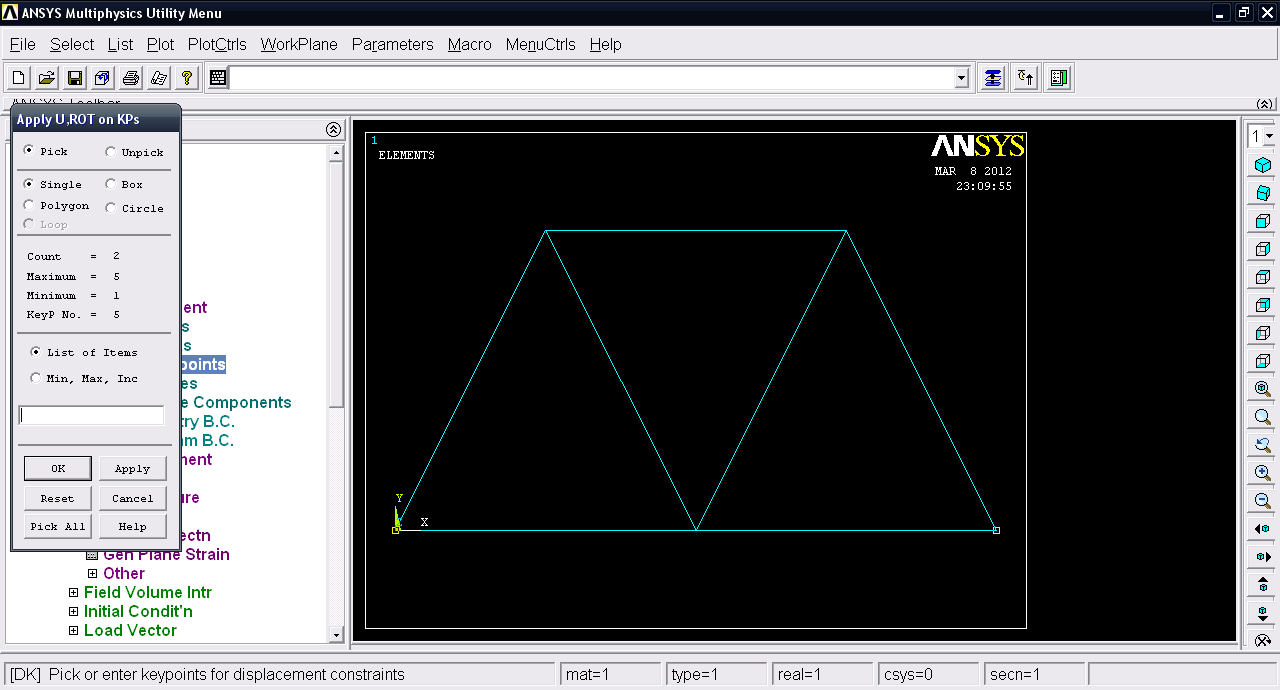4. ### Step 4:

Select All DOF and click OK.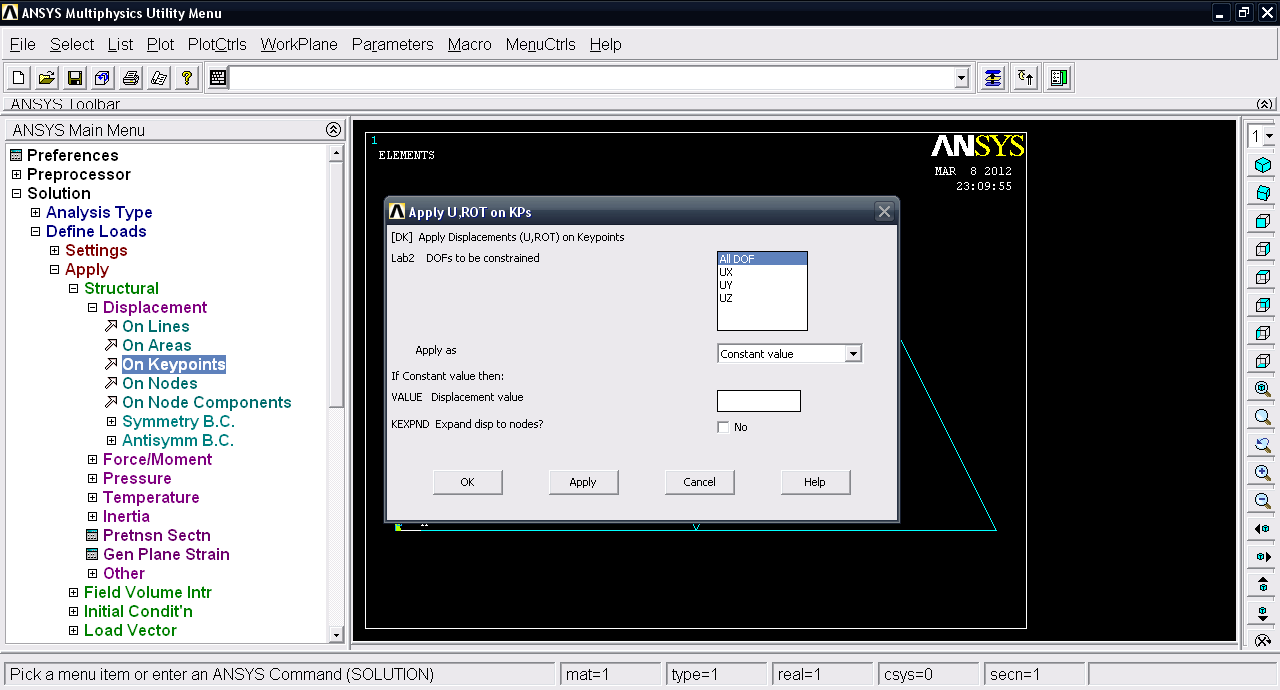5. ### Step 5:

Goto Define loads >> Apply >> Structural >> Force/Moment >> On Keypoints.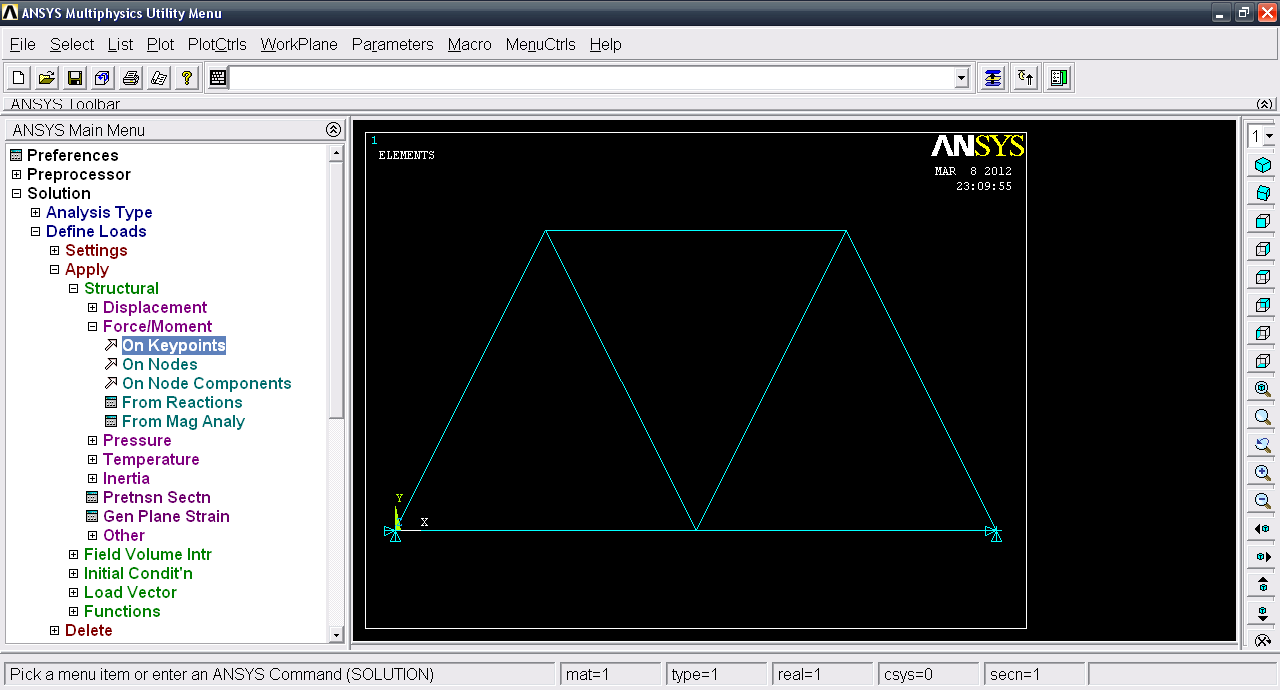6. ### Step 6:

Select the upper keypoints and click OK.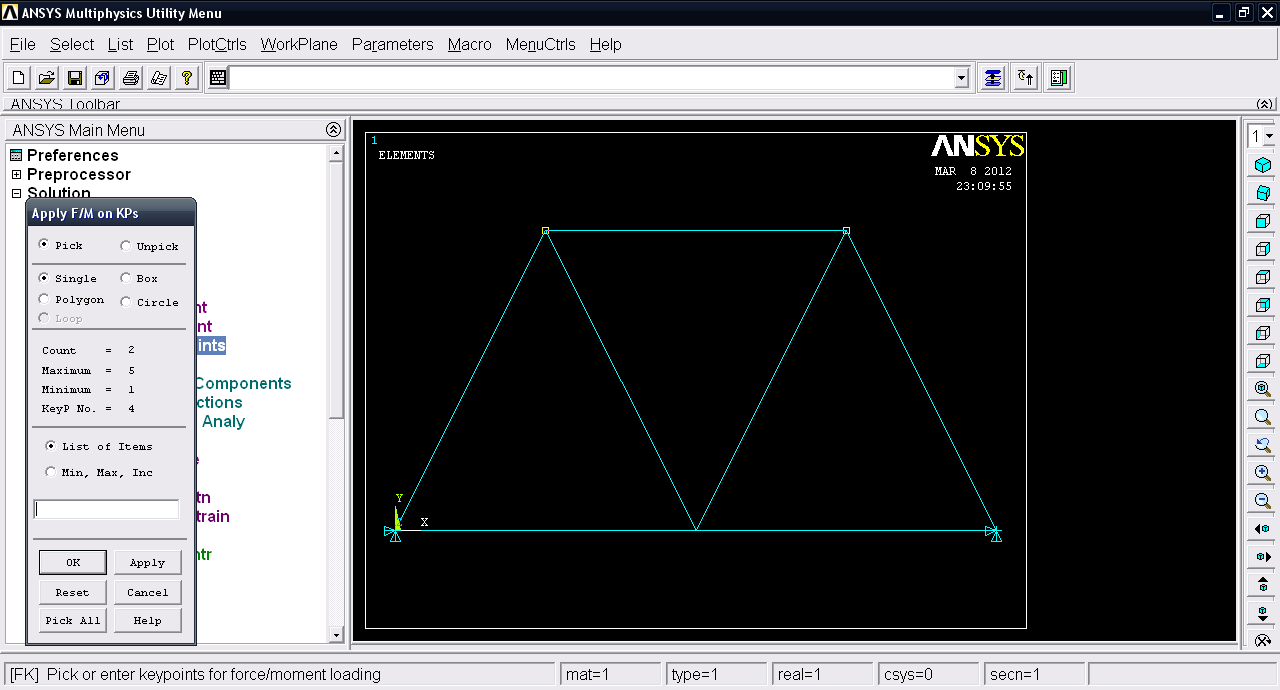7. ### Step 7:

Direction of force be FY and input Force value = -10000 since the force will be acting downward.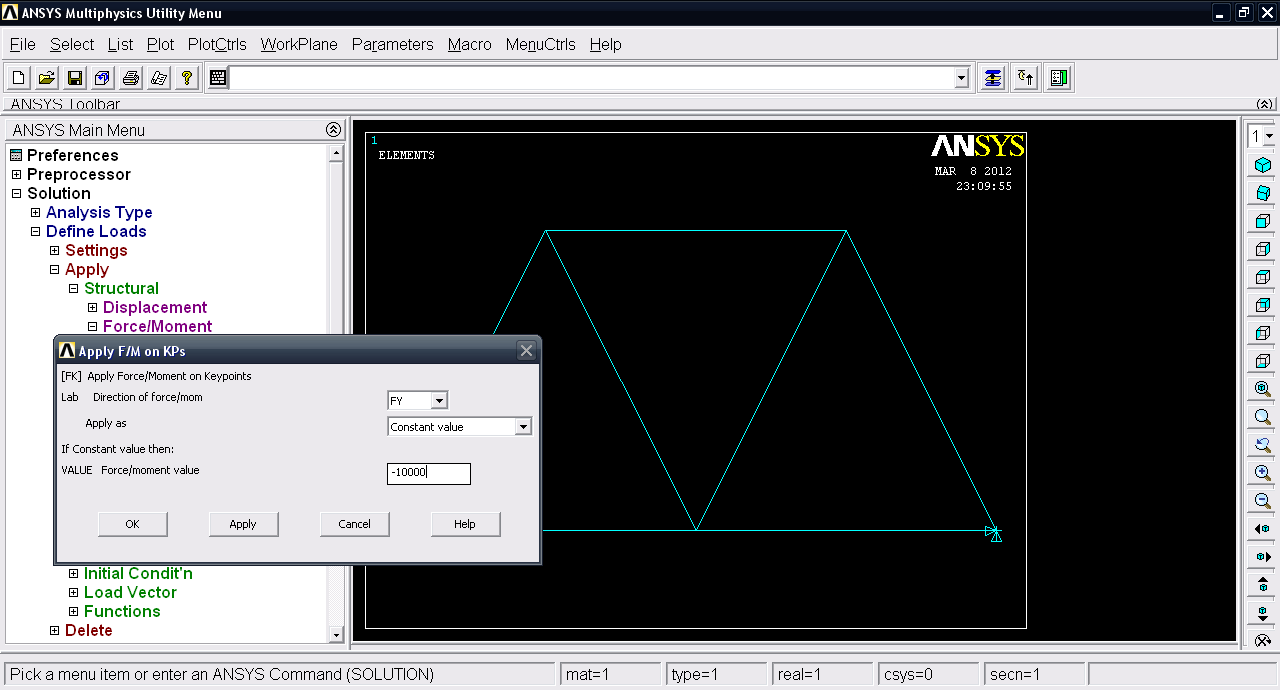8. ### Step 8:

Now we have the model prepared for solution. Under Solve >> Current Load step.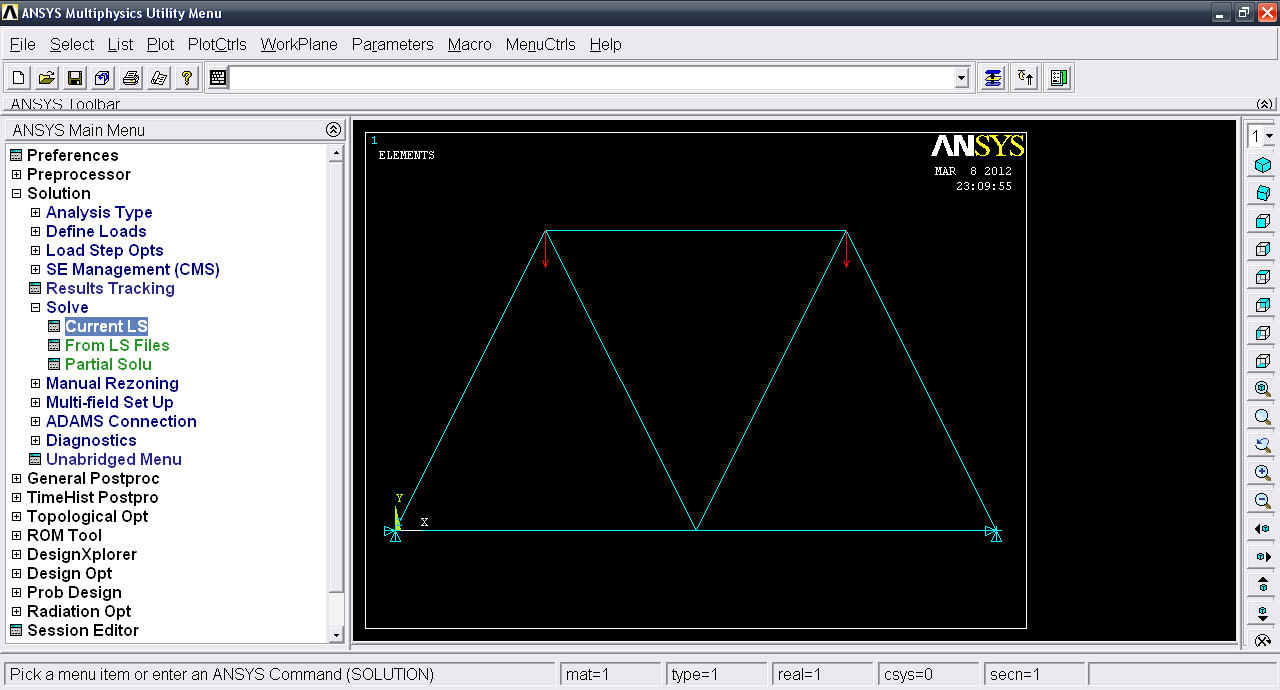9. ### Step 9:

Click OK.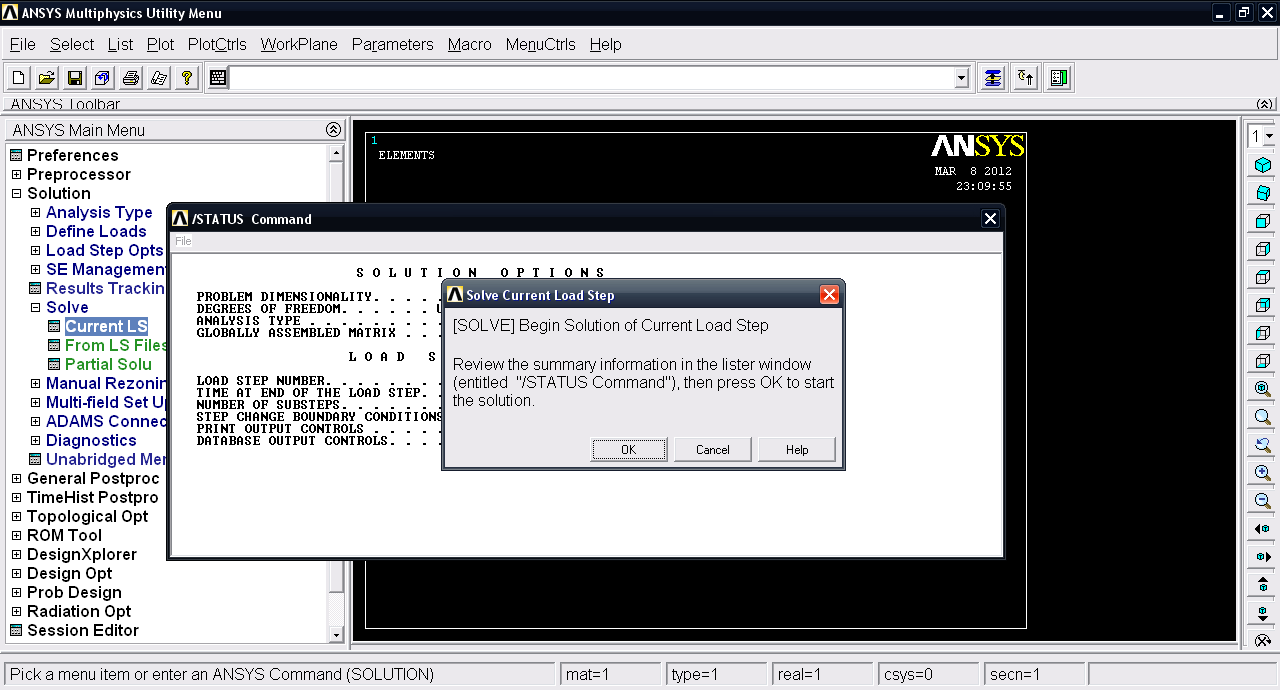10. ### Step 10:

A message Solution is done! will show when the process is completed. Click Close.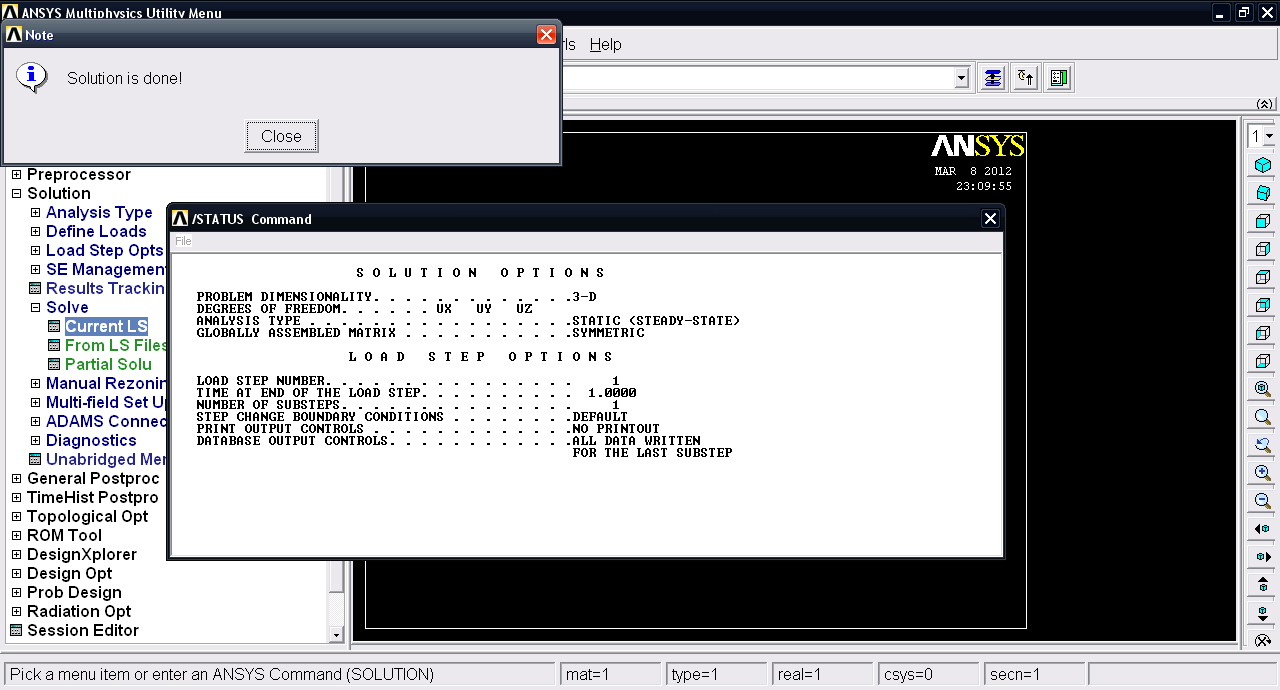11. ### Step 11:

Now the third part of this process. Post-Processing is to be done. Goto General PostProc >> List Results >> Reaction Solu.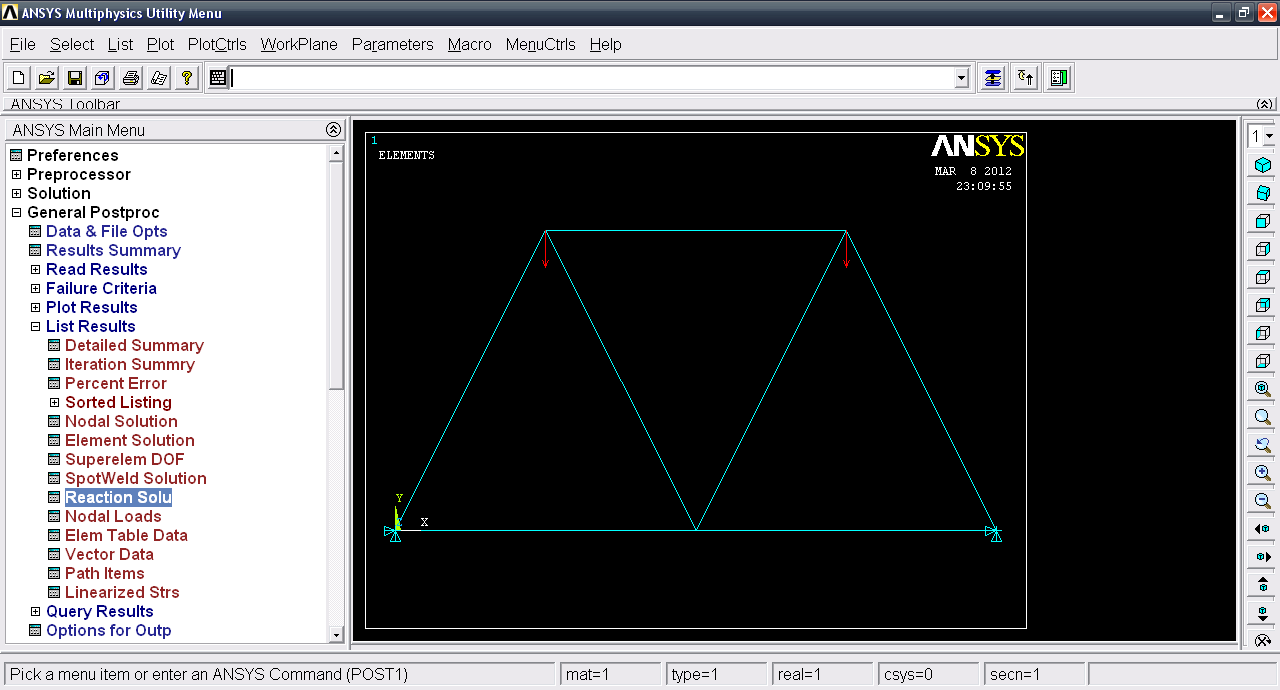12. ### Step 12:

Select all items and click OK.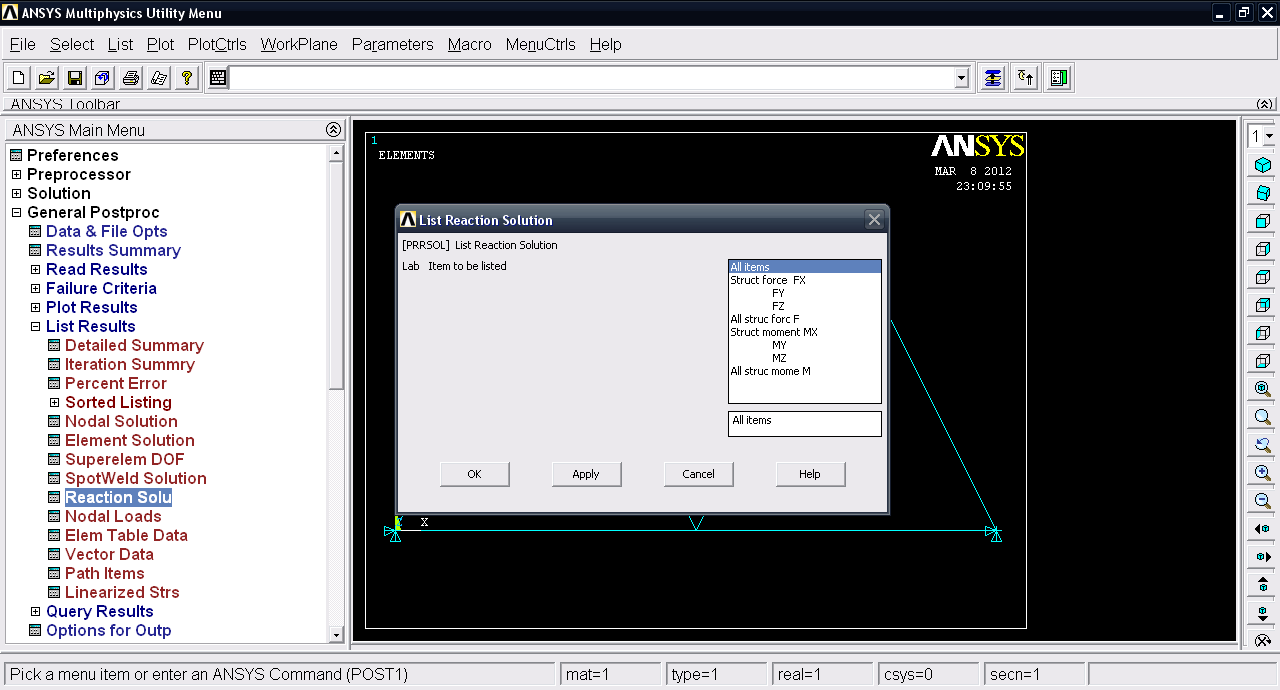13. ### Step 13:

Now we have the value of reaction about the node 1 and 5 which are fixed. Click Close.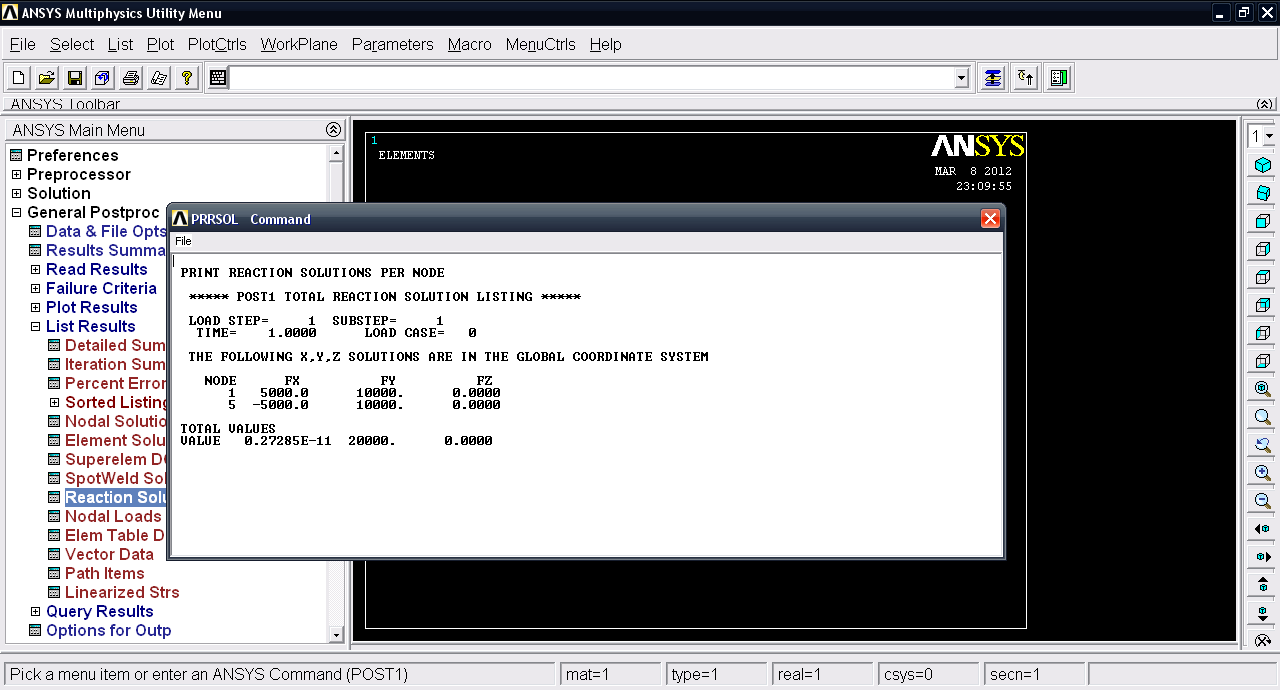14. ### Step 14:

Goto Plot Results >> Deformed shape.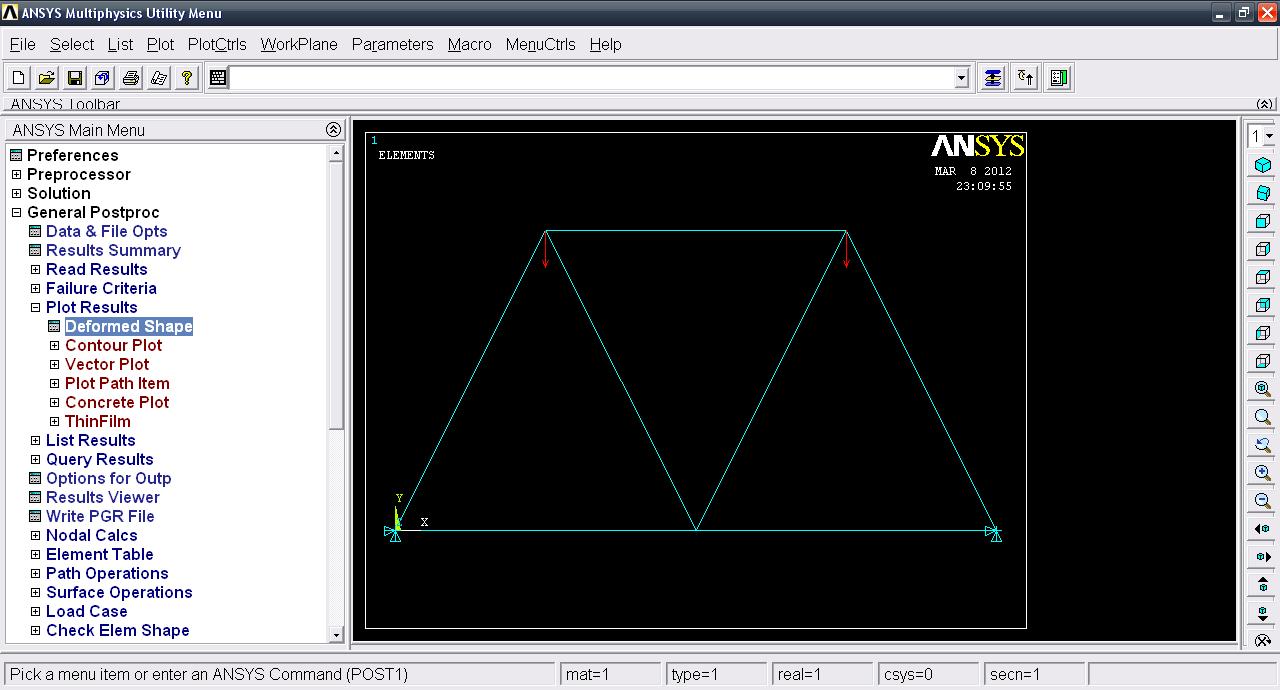15. ### Step 15:

Select Def + undeformed. Click OK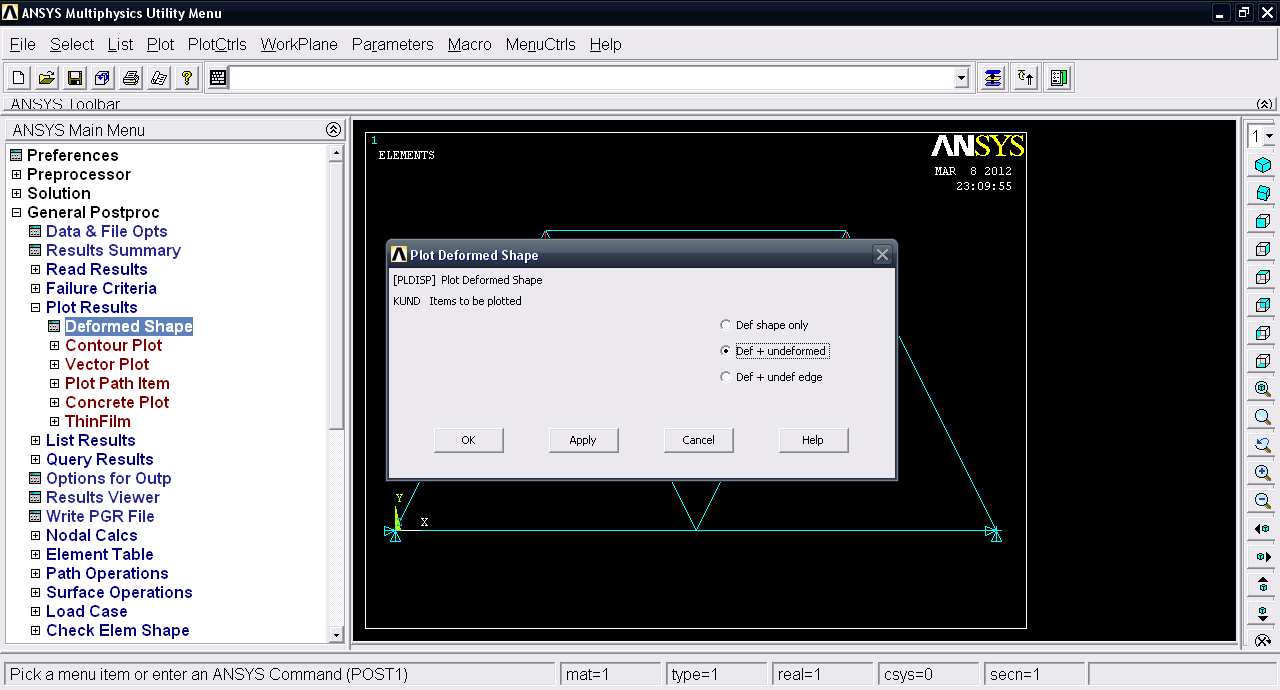16. ### Step 16:

Now we have the deformation plot.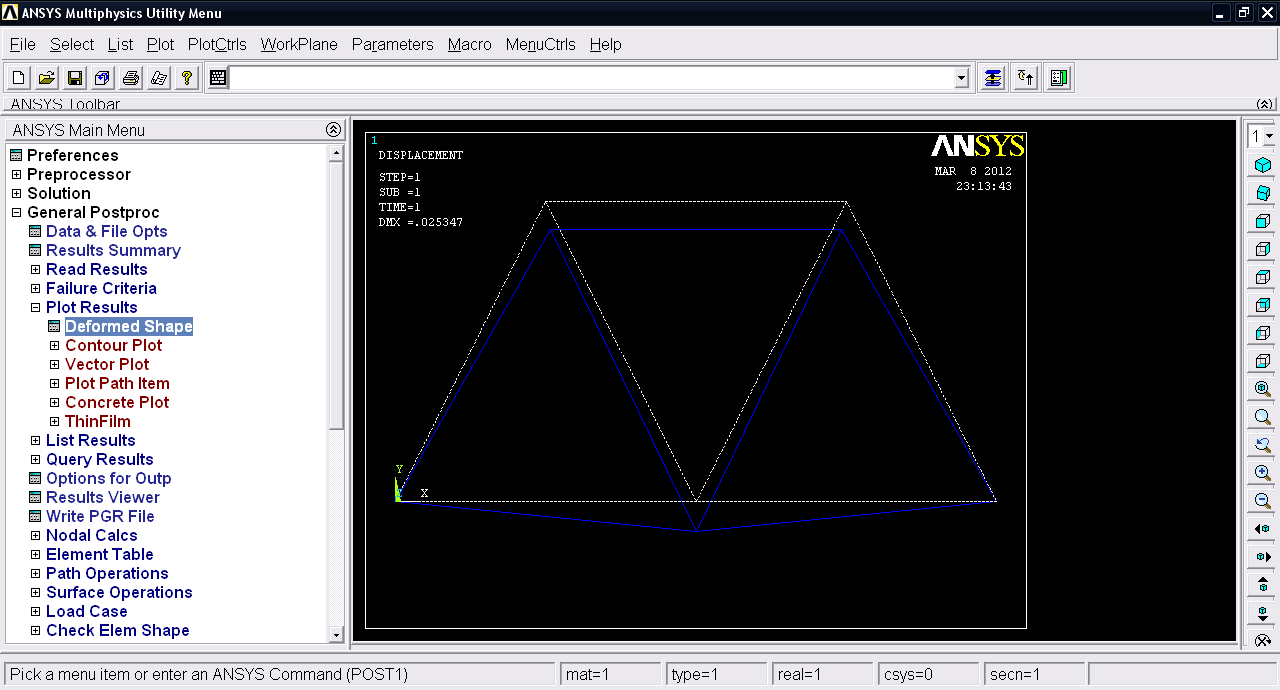17. ### Step 17:

Goto Plot results >> Contour plot >> Nodal Solu.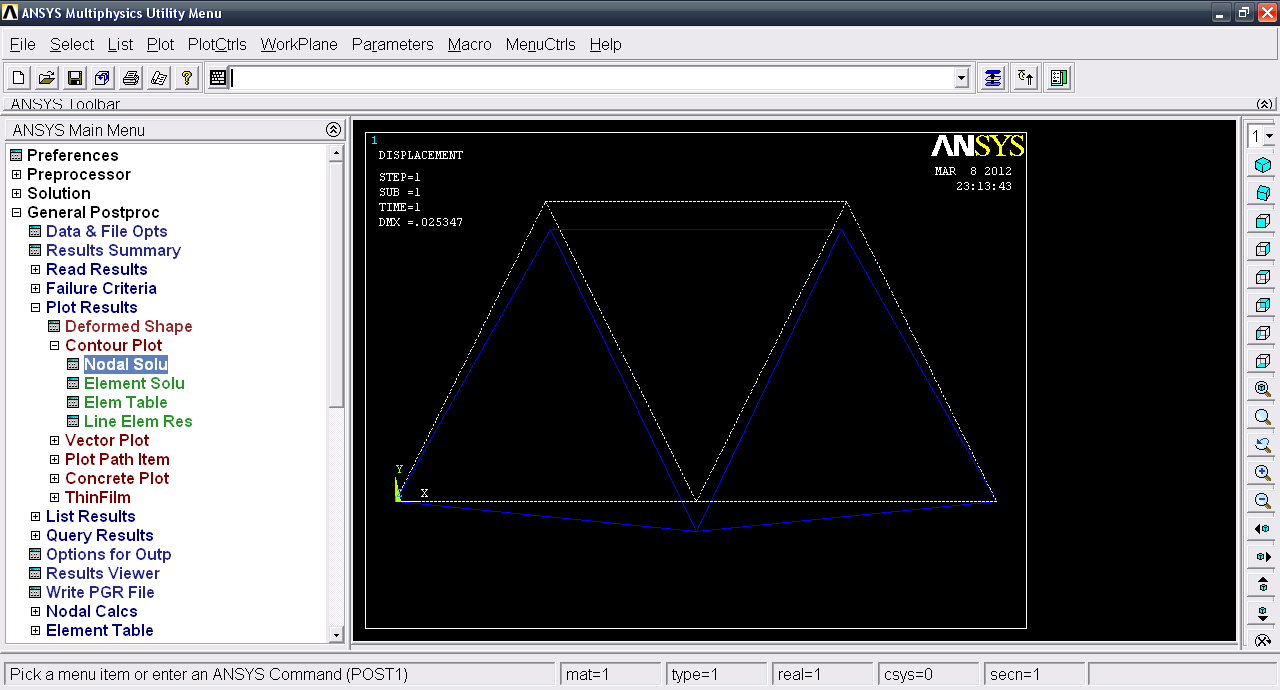18. ### Step 18:

Under Stress select von Mises stress and click OK.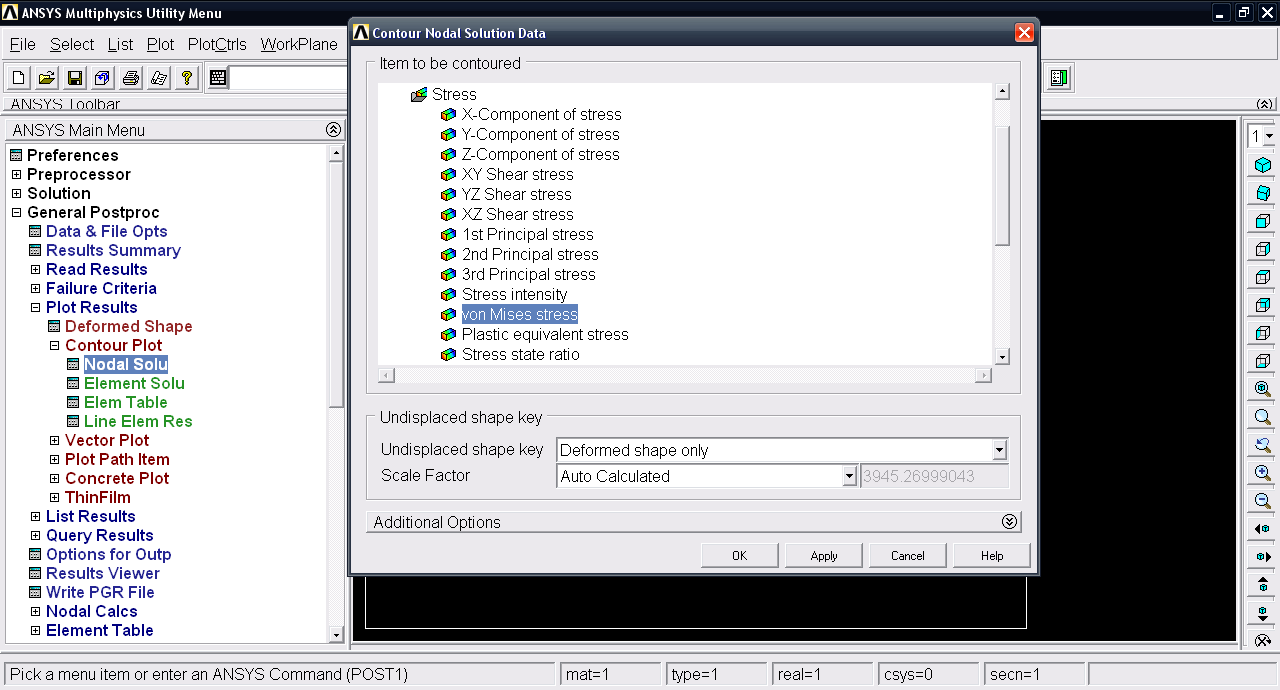19. ### Step 19:

We have von Mises stress plot.